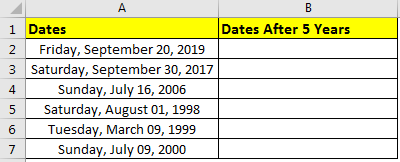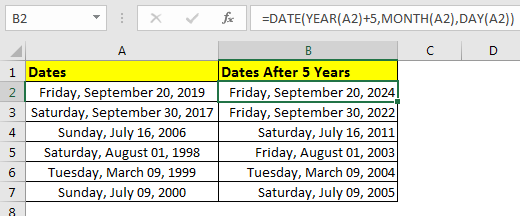# How to Add Years to a Date in Excel

If you want to add years to a date in excel, you can’t just simply do an addition to add years. If you add 1 year as a number to a date, it wil add 1 day. In excel, 1 is equal to 1 day (24 hours). If you add multiples of 365 to a given date then it will give an incorrect answer, since there can be a leap year in between. To add years to a date in excel, we use a formula.

Generic Formula

=DATE(YEAR(date)+num_years, MONTH(date),DAY(date))

Date: the date in which you want to add years.
Num_years: the number of years you want to add.
Example: Add 5 Years to Start Date.
Here we have some dates. In each date we need to add five years.Write this formula in cell B2 and copy it down the rows.

=DATE(YEAR(A2)+5,MONTH(A2),DAY(A2))

This returns a date 5 years ahead of start date.How it works?
It’s simple. We extracted year, month and date from date using function of same name. Added 5 to years YEAR(A2)+5. And then integrated year, month and date using DATE function of excel. This gave us a new date of 5 years ahead.
How to subtract years from date?
Just replace the addition sign with subtraction sign (“-”) subtract years from a date.

=DATE(YEAR(A2)-5,MONTH(A2),DAY(A2))

Above formula will give you a date exact 5 years before.

So yeah guys, this how you can add years to a date in excel easily. In example I have used hardcoded years. You can also give reference of cell so that it can be dynamic. Let me know if you have any query regarding this or any other topic of Excel and VBA.

Popular Articles

50 Excel Shortcut to Increase Your Productivity: Get faster at your task. These 50 shortcuts will make you work even faster on Excel.

How to use the VLOOKUP Function in Excel: This is one of the most used and popular functions of excel that is used to lookup value from different ranges and sheets.

How to use the COUNTIF function in Excel: Count values with conditions using this amazing function. You don't need to filter your data to count specific values. Countif function is essential to prepare your dashboard.

How to use the SUMIF Function in Excel: This is another dashboard essential function. This helps you sum up values on specific conditions.

Terms and Conditions of use

The applications/code on this site are distributed as is and without warranties or liability. In no event shall the owner of the copyrights, or the authors of the applications/code be liable for any loss of profit, any problems or any damage resulting from the use or evaluation of the applications/code.